##Advanced Graphics Study Guide

Advanced Graphics, Dr Neil Dodgson, University of Cambridge Computer Laboratory
Part II course, 2000

Part 2: Geometric primitives
A: Ray tracing primitives
B: Conics, quadrics and superquadrics
...back to part 1 | on to part 3...

# 2A) Ray tracing primitives

Relevant mainly to RT.

A primitive is a shape for which a ray-shape intersection routine has been written. More complex objects can be built out of the primitives. Most ray tracers will have a variety of primitives. They are limited only by the ability of the programmer to write a function to analytically intersect a ray with the surface of the shape.

## Common primitives

Plane
The infinite plane is a simple object with which to intersect a ray (see Part IB notes for details). On its own it can represent boundary objects such as the ground or the sky or perhaps an infinite wall. Intersection with the infinite plane is a useful building block in a ray tracing system.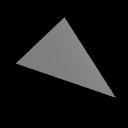Polygon
Having the polygon as a ray tracing primitive allows a ray tracer to render anything that a PSC algorithm could. To find the intersection of a ray with a polygon, first find the intersection of the ray with the infinite plane in which the polygon lies. Then ascertain whether the intersection lies inside or outside the polygon: this is a reasonably straightforward two dimensional graphics operation.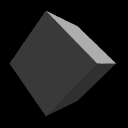Box
A box is essentially six polygons and could be ray traced as such. However, intersection with an axis-aligned box can be optimised. Any box can be axis-aligned by appropriate transformations. We can thus write a routine to intersect an arbitrary ray with an axis-aligned box and then transform the ray under consideration in exactly the same way as we transform the box which we are trying to intersect with it. This sort of idea generalises neatly to the concept of specifying any object in a convenient object coordinate system and then applying transforms to the whole object to place it at the appropriate point in the world coordinate system.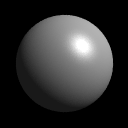Sphere
The sphere is the simplest finite object with which to intersect a ray. Practically any ray tracing program will include the sphere as a primitive. Scaling a sphere by different amounts along the different axes will produce an ellipsoid: a squashed or stretched sphere. There is thus no need to include the ellipsoid as a primitive provided that your ray tracer contains the usual complement of transformations. (It would be a poor ray tracer if it did not!)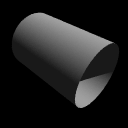Cylinder
Intersecting a ray with an infinitely long cylinder is practically as easy as intersecting one with a sphere. The tricky bit, if it can be called that, is to intersect a ray with a more useful finite length cylinder. This is achieved by intersecting the ray with the appropriate infinitely long cylinder and then ascertaining where along the cylinder the intersection lies. If it lies in the finite length in which you are interested then keep the intersection. If it does not then ignore the intersection. Note that the ray tracer used to render the accompanying image has cylinders without end caps. This is the correct result if you follow the procedure outlined in this paragraph. Adding end caps to your cylinders requires extra calculations.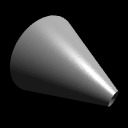Cone
Cones are very like cylinders. Like the infinite cylinder, there is a simple mathematical definition of an infinite cone which makes it easy to write a ray-cone intersection algorithm. Note that a cone does not need to have a point -- it can be truncated short of its `top', as illustrated in the accompanying image. The particular ray tracer used does not add end caps to cones.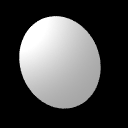Disc
The disc is not a common ray tracing primitive, but is necessary in ray tracers which implement cones and cylinders without end caps. It is handled in much the same way as the polygon. The routine to check if the intersection with the plane lies inside or outside of the disc is simpler than the equivalent routine for the polygon. In the accompanying ray traced image, the disc has been positioned so that it just catches the light -- this illustrates how specular reflection varies across a completely flat surface. The sphere, cone and torus images illustrate how specular reflection varies across three curved surfaces.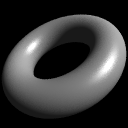Torus
Toroids are reasonably rare in real life (doughnuts and tyre inner tubes notwithstanding). They are somehow alluring to the kinds of people who implement ray tracers and, having a reasonably straightforward mathematical definition, are reasonably simple to implement. They thus appear as primitives in many ray tracers. They become more useful when combined with Constructive Solid Geometry (see Part 4C).

## Ray-primitive intersections

SMEG section 1 contains the mathematics of ray-primitive intersections and details of how you would go about converting a primitive object into polygons for PSC.

 Exercises Work out mathematical equations to define a plane, a sphere, an infinitely long cylinder, an infinitely long cone, and a torus. You will find it helpful to centre each primitive at the origin and to align it in a sensible way with respect to the coordinate axes. You may like to use cylindrical polar coordinates where these prove useful. As a starting point remember that a circle can be represented by the equation: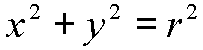Work out a way to intersect a ray with each of the five primitives. You may assume that you are provided with functions to find the roots of linear, quadratic, cubic and quartic equations. Work out if there exists a faster intersection algorithm for an axis aligned 2x2x2 unit box than just six separate polygon intersection calculations. [1999/7/11] (a) Give a parametric definition of a torus centred at the origin and aligned with the coordinate axes. (b) Outline how you would find the first intersection point, if any, of a ray with the torus from the previous part. Show how to convert a cylinder into a polygon mesh. What changes do you have to make if the mesh may contain only triangles? Show how to convert a torus into a polygon mesh. Show how to convert a sphere into a triangle mesh. How can you get the most even distribution of triangle vertices across the sphere? [2000/9/4] (a) Show how you would calculate the first intersection point between an arbitrary ray and a finite length open cylinder of unit radius aligned along the \$x\$-axis. [Note: an open cylinder is one which has no end caps.] Having found the intersection point, how would you find the normal vector?

Part 2: Geometric primitives
A: Ray tracing primitives
B: Conics, quadrics and superquadrics
...back to part 1 | on to part 3...

Neil Dodgson | Advanced Graphics | Computer Laboratory

Source file: p2a.html
Page last updated on Thu Sep 14 16:58:04 BST 2000
by Neil Dodgson (nad@cl.cam.ac.uk)